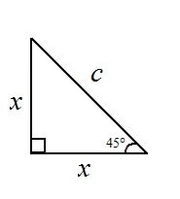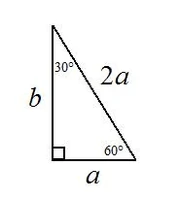38 Pages

A triangle is any polygon with three sides.

## Properties

The sum of the angles in any triangle must always be equal to 180°. Radians are not used to measure triangle angles, since they only apply to circles.

The area of any given triangle is described in this formula:where b is the base of the triangle and h is the height of the triangle.

Hero of Alexandria is credited with the following formula. If a triangle has sides a, b, and c:where s is the semiperimeter of the triangle, i.e. the sum of the sides divided by 2. This formula does not require knowledge of the triangle's height.

## Triangle Classifications Based On Angles

### Right

A right triangle is any triangle with an angle of 90°.

#### Special Right Triangles

##### 45-45-90A 45-45-90 triangle with sides labeled.

A 45-45-90 triangle is a triangle whose angles measure only 90° and 45°. Shortcuts to finding the lengths of missing sides can be found here.,

so therefore,, or##### 30-60-90A 30-60-90 triangle with sides labeled. Note the absence of c.

A 30-60-90 triangle is a triangle whose angles measure only 30°, 60°, and 90°. Shortcuts to finding the lengths of missing sides can be found here.,

so therefore,, or### Acute

An acute triangle is any triangle where all the angles measure less than 90°.

#### Equiangular

An equiangular triangle is an acute triangle in which all sides and angles are the same. The angles in equiangular triangles always measure 60°. This is true because 180° ÷ 3 = 60°.

### Obtuse

An obtuse triangle is any triangle where one angle measures more than 90°.

## Triangle Classification Based On Sides

### Equilateral

An equilateral (Latin aequalis "equal" + latus "side") triangle, also called an equiangular triangle, is an acute triangle in which all sides and angles are the same. The angles in equilateral triangles always measure 60°. This is true because 180° ÷ 3 = 60°.

### Isosceles

An isosceles (Greek ίσος ísos "same" + σκελες skeles "legs") triangle is any triangle where 2 sides are congruent, leaving the base angles congruent as well.

### Scalene

A scalene triangle is any triangle where no sides are congruent.

Community content is available under CC-BY-SA unless otherwise noted.